Скачать презентацию Secure Multiparty Computation Ivan Damgård Department of Computer

78553d4e272ede71f9244deefe6d44ad.ppt

• Количество слайдов: 42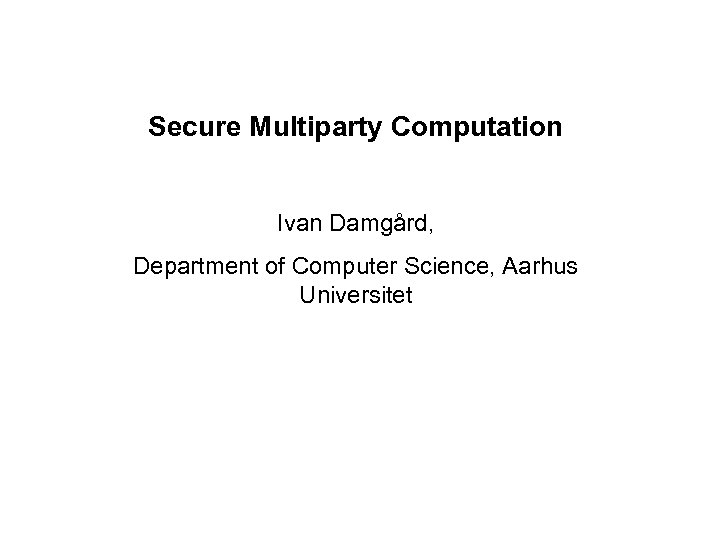Secure Multiparty Computation Ivan Damgård, Department of Computer Science, Aarhus UniversitetPrivate Information PIN codes, Customer database of a company, patient journals in a hospital, . . - Valuable information must be confidential, and must not be lost. - But often ADDED value can be obtained by combining private data from different sources - A few examples. . .Simple ”first price” Auction Participants bid for an item, highest bid wins. Each player has private information: the max price you are willing to pay. Of course everyone want to pay as little as possible Goal: find winner and price to pay in a fair way.Dobbele Auktion – an “Exchange” Many sellers and buyers want to trade different quantities of some commodity. Each seller is willing to sell different quantities, depending on the price. Likewise for buyers Goal find a fair market price, given supply and demand in the market, in a fair way. For instance, maximal price you want to buy at must remain private.Benchmarking Some companies working in the same sector have data describing how well business is going: production costs, turnover, salaries, etc. Goal: each company wants to find out how well it is doing in comparison to the others. - But of course without revealing information to competitors. .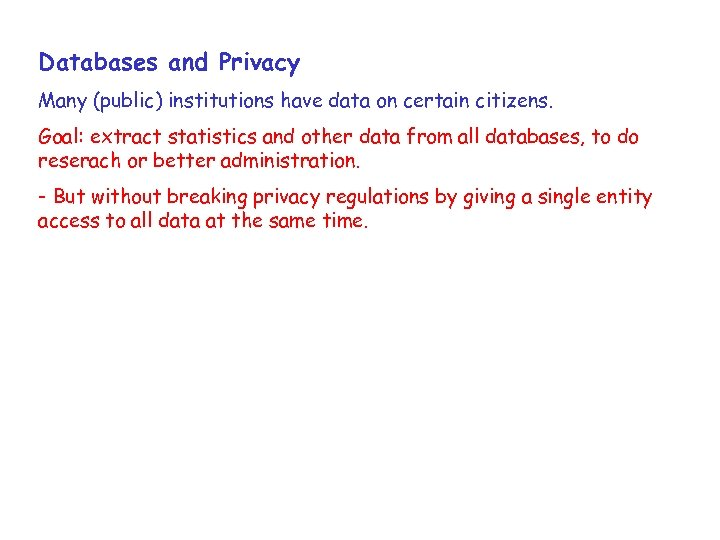Databases and Privacy Many (public) institutions have data on certain citizens. Goal: extract statistics and other data from all databases, to do reserach or better administration. - But without breaking privacy regulations by giving a single entity access to all data at the same time.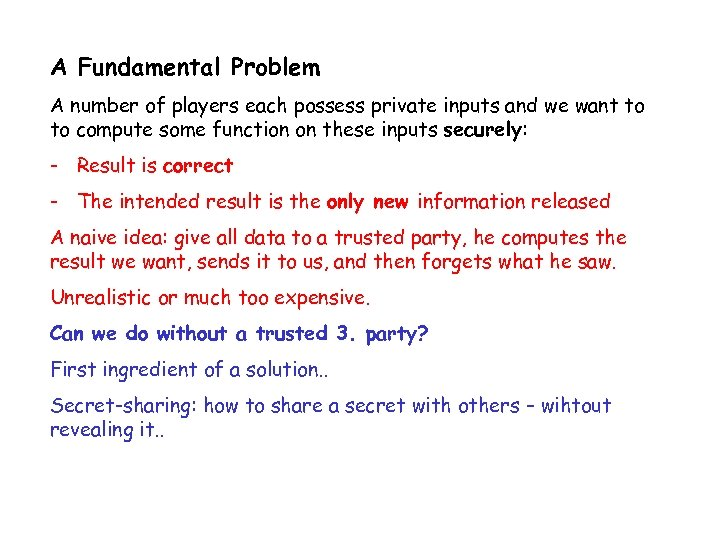A Fundamental Problem A number of players each possess private inputs and we want to to compute some function on these inputs securely: - Result is correct - The intended result is the only new information released A naive idea: give all data to a trusted party, he computes the result we want, sends it to us, and then forgets what he saw. Unrealistic or much too expensive. Can we do without a trusted 3. party? First ingredient of a solution. . Secret-sharing: how to share a secret with others – wihtout revealing it. .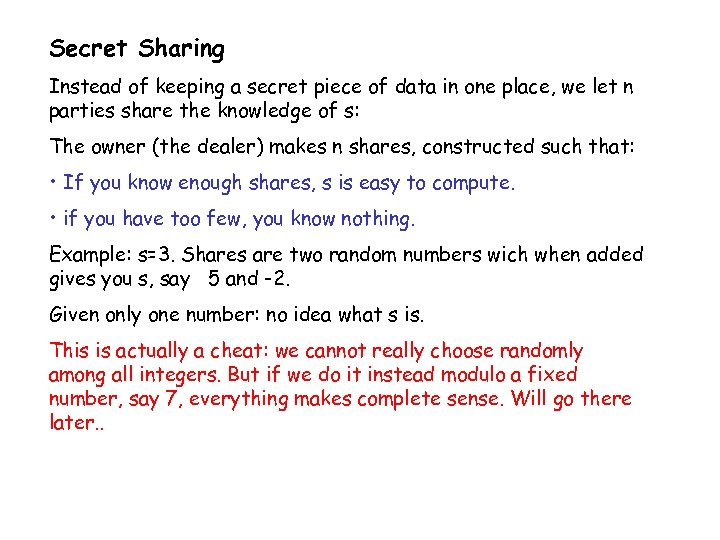Secret Sharing Instead of keeping a secret piece of data in one place, we let n parties share the knowledge of s: The owner (the dealer) makes n shares, constructed such that: • If you know enough shares, s is easy to compute. • if you have too few, you know nothing. Example: s=3. Shares are two random numbers wich when added gives you s, say 5 and -2. Given only one number: no idea what s is. This is actually a cheat: we cannot really choose randomly among all integers. But if we do it instead modulo a fixed number, say 7, everything makes complete sense. Will go there later. .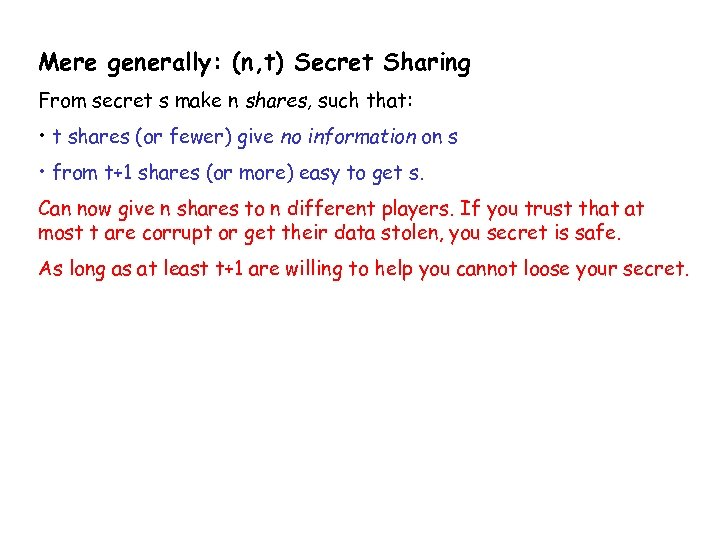Mere generally: (n, t) Secret Sharing From secret s make n shares, such that: • t shares (or fewer) give no information on s • from t+1 shares (or more) easy to get s. Can now give n shares to n different players. If you trust that at most t are corrupt or get their data stolen, you secret is safe. As long as at least t+1 are willing to help you cannot loose your secret.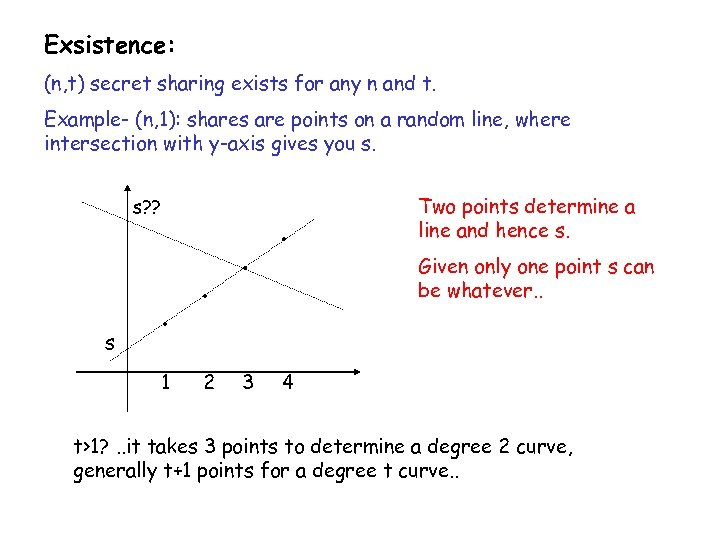Exsistence: (n, t) secret sharing exists for any n and t. Example- (n, 1): shares are points on a random line, where intersection with y-axis gives you s. s? ? s · 1 · 2 · 3 · Two points determine a line and hence s. Given only one point s can be whatever. . 4 t>1? . . it takes 3 points to determine a degree 2 curve, generally t+1 points for a degree t curve. .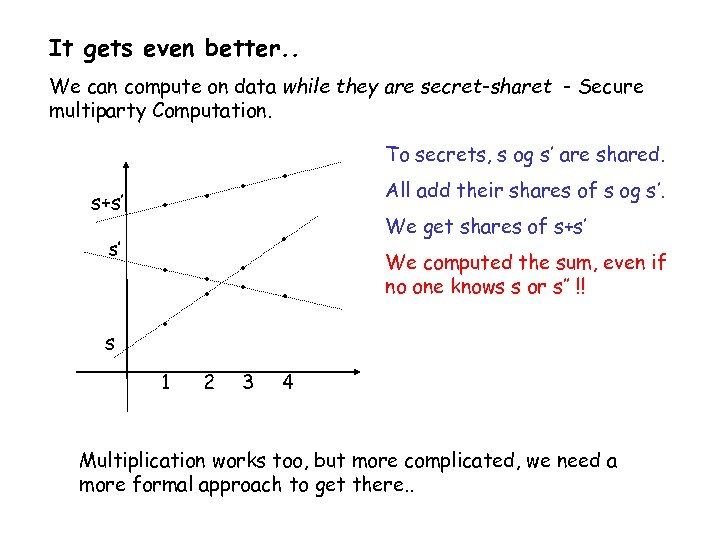It gets even better. . We can compute on data while they are secret-sharet - Secure multiparty Computation. s+s’ s’ s · · · 1 2 3 To secrets, s og s’ are shared. All add their shares of s og s’. We get shares of s+s’ We computed the sum, even if no one knows s or s’’ !! 4 Multiplication works too, but more complicated, we need a more formal approach to get there. .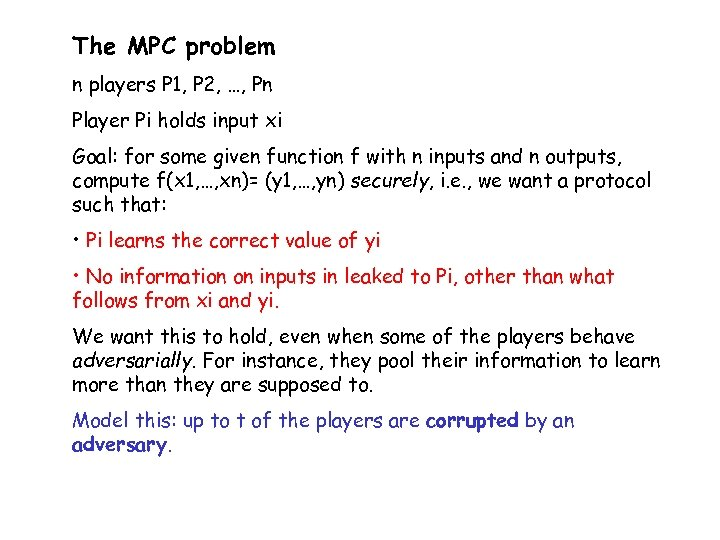The MPC problem n players P 1, P 2, …, Pn Player Pi holds input xi Goal: for some given function f with n inputs and n outputs, compute f(x 1, …, xn)= (y 1, …, yn) securely, i. e. , we want a protocol such that: • Pi learns the correct value of yi • No information on inputs in leaked to Pi, other than what follows from xi and yi. We want this to hold, even when some of the players behave adversarially. For instance, they pool their information to learn more than they are supposed to. Model this: up to t of the players are corrupted by an adversary.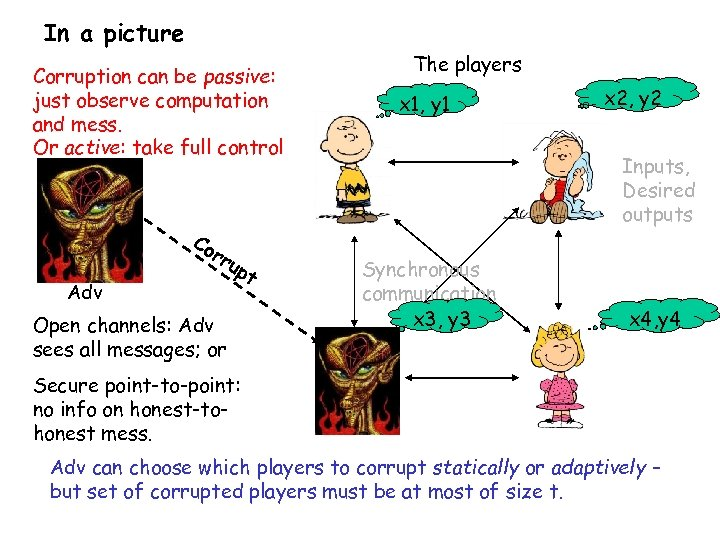In a picture Corruption can be passive: just observe computation and mess. Or active: take full control Co Adv rr up Open channels: Adv sees all messages; or t The players x 1, y 1 x 2, y 2 Inputs, Desired outputs Synchronous communication x 3, y 3 x 4, y 4 Secure point-to-point: no info on honest-tohonest mess. Adv can choose which players to corrupt statically or adaptively – but set of corrupted players must be at most of size t.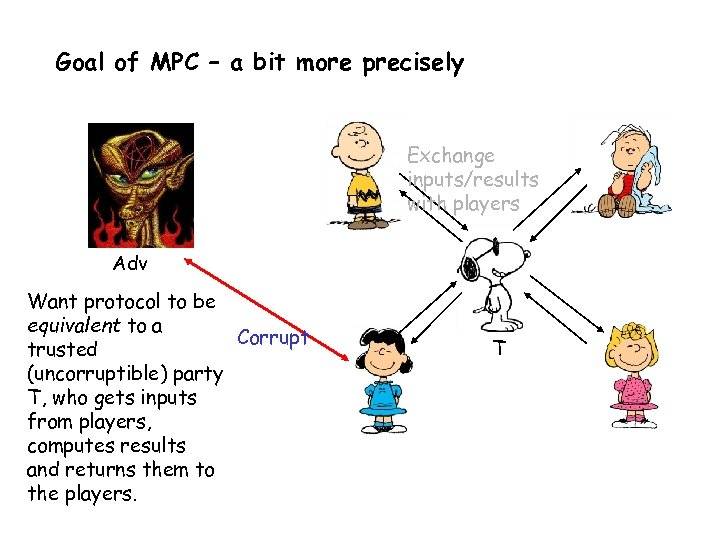Goal of MPC – a bit more precisely Exchange inputs/results with players Adv Want protocol to be equivalent to a Corrupt trusted (uncorruptible) party T, who gets inputs from players, computes results and returns them to the players. T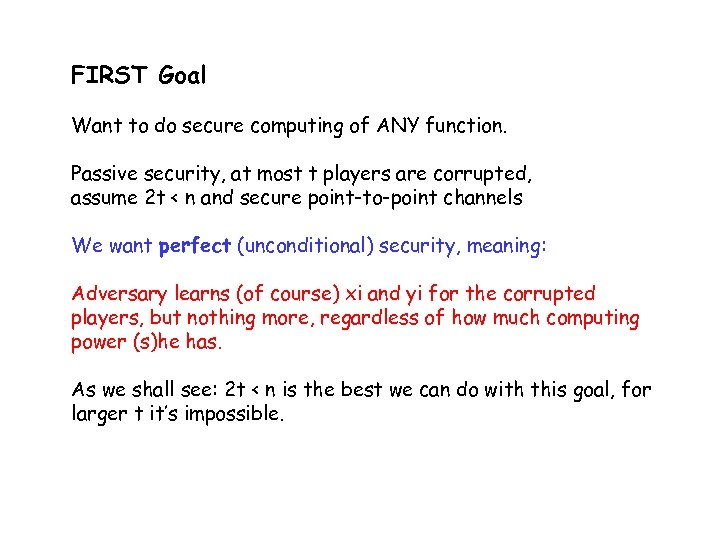FIRST Goal Want to do secure computing of ANY function. Passive security, at most t players are corrupted, assume 2 t < n and secure point-to-point channels We want perfect (unconditional) security, meaning: Adversary learns (of course) xi and yi for the corrupted players, but nothing more, regardless of how much computing power (s)he has. As we shall see: 2 t < n is the best we can do with this goal, for larger t it’s impossible.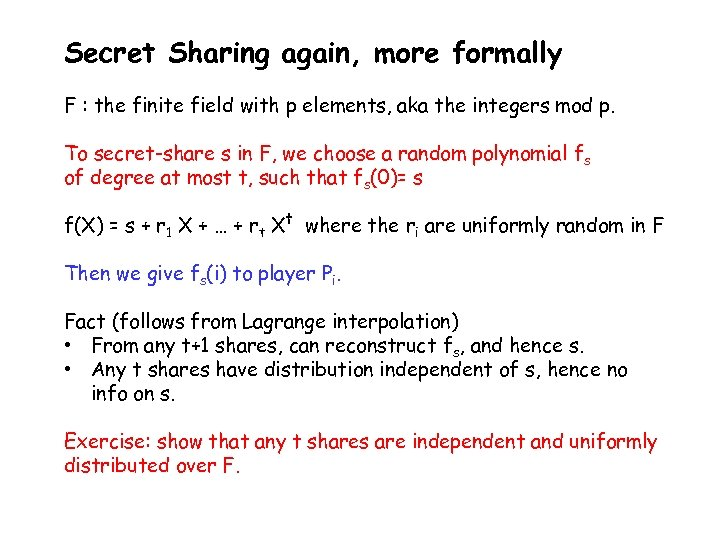Secret Sharing again, more formally F : the finite field with p elements, aka the integers mod p. To secret-share s in F, we choose a random polynomial fs of degree at most t, such that fs(0)= s f(X) = s + r 1 X + … + rt Xt where the ri are uniformly random in F Then we give fs(i) to player Pi. Fact (follows from Lagrange interpolation) • From any t+1 shares, can reconstruct fs, and hence s. • Any t shares have distribution independent of s, hence no info on s. Exercise: show that any t shares are independent and uniformly distributed over F.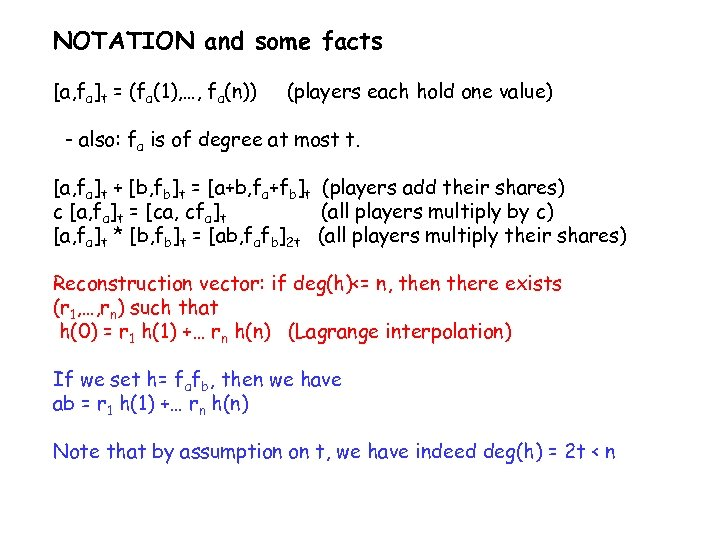NOTATION and some facts [a, fa]t = (fa(1), …, fa(n)) (players each hold one value) - also: fa is of degree at most t. [a, fa]t + [b, fb]t = [a+b, fa+fb]t (players add their shares) c [a, fa]t = [ca, cfa]t (all players multiply by c) [a, fa]t * [b, fb]t = [ab, fafb]2 t (all players multiply their shares) Reconstruction vector: if deg(h)<= n, then there exists (r 1, …, rn) such that h(0) = r 1 h(1) +… rn h(n) (Lagrange interpolation) If we set h= fafb, then we have ab = r 1 h(1) +… rn h(n) Note that by assumption on t, we have indeed deg(h) = 2 t < n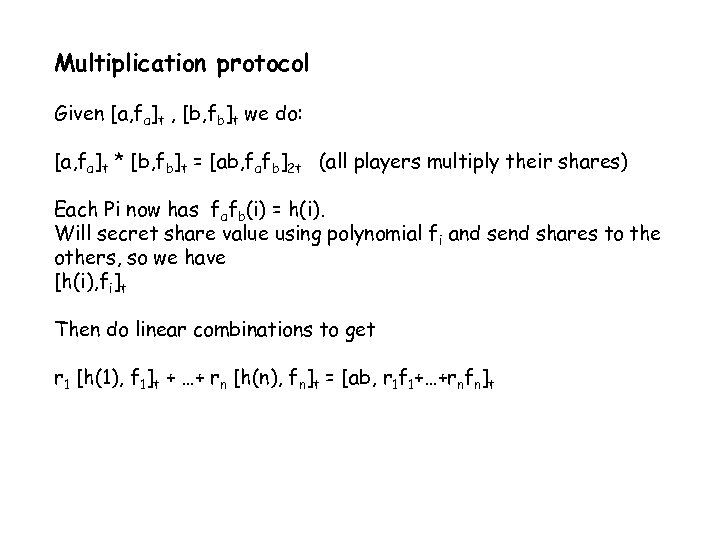Multiplication protocol Given [a, fa]t , [b, fb]t we do: [a, fa]t * [b, fb]t = [ab, fafb]2 t (all players multiply their shares) Each Pi now has fafb(i) = h(i). Will secret share value using polynomial fi and send shares to the others, so we have [h(i), fi]t Then do linear combinations to get r 1 [h(1), f 1]t + …+ rn [h(n), fn]t = [ab, r 1 f 1+…+rnfn]t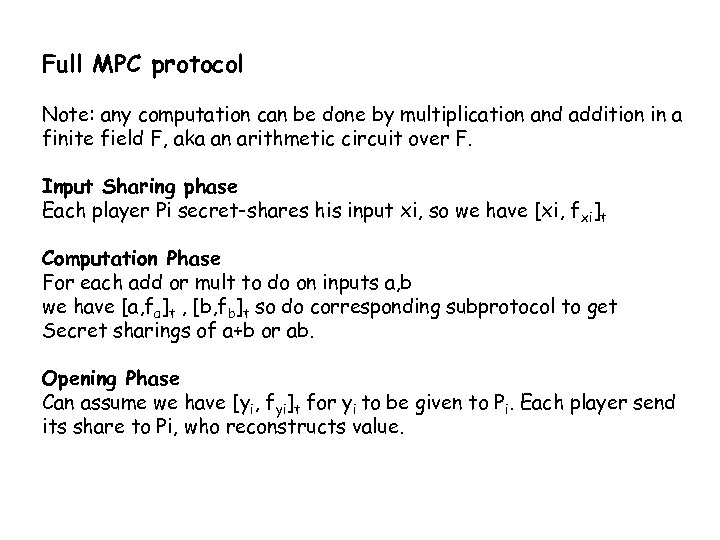Full MPC protocol Note: any computation can be done by multiplication and addition in a finite field F, aka an arithmetic circuit over F. Input Sharing phase Each player Pi secret-shares his input xi, so we have [xi, f xi]t Computation Phase For each add or mult to do on inputs a, b we have [a, fa]t , [b, fb]t so do corresponding subprotocol to get Secret sharings of a+b or ab. Opening Phase Can assume we have [yi, fyi]t for yi to be given to Pi. Each player send its share to Pi, who reconstructs value.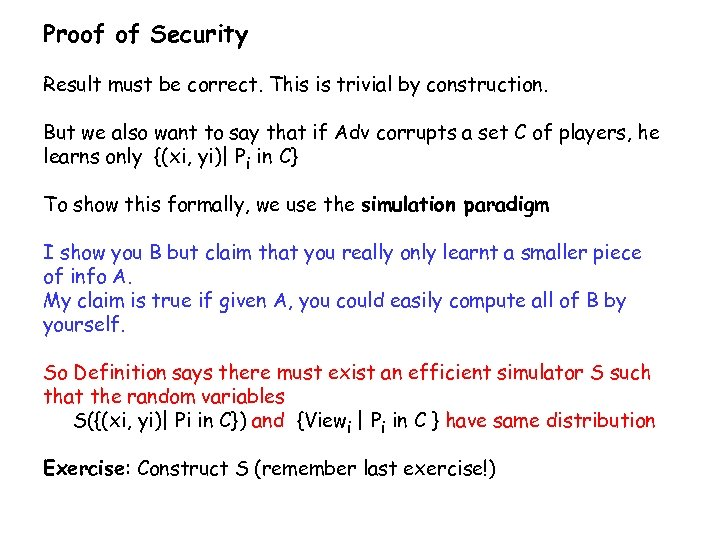Proof of Security Result must be correct. This is trivial by construction. But we also want to say that if Adv corrupts a set C of players, he learns only {(xi, yi)| Pi in C} To show this formally, we use the simulation paradigm I show you B but claim that you really only learnt a smaller piece of info A. My claim is true if given A, you could easily compute all of B by yourself. So Definition says there must exist an efficient simulator S such that the random variables S({(xi, yi)| Pi in C}) and {Viewi | Pi in C } have same distribution Exercise: Construct S (remember last exercise!)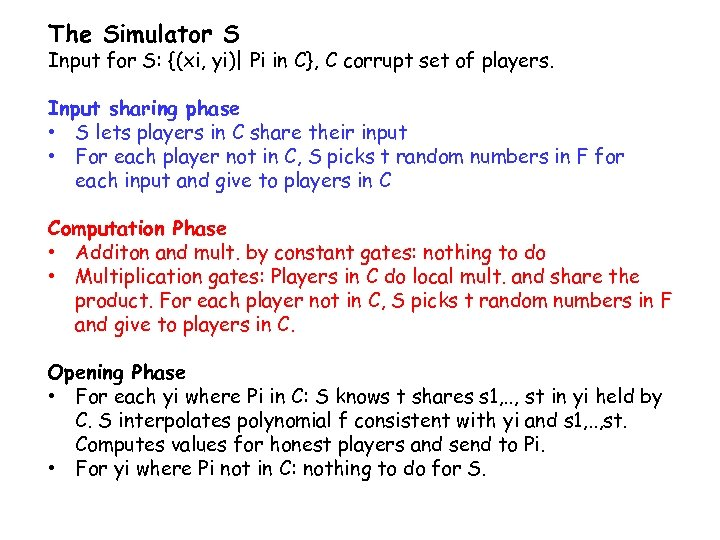The Simulator S Input for S: {(xi, yi)| Pi in C}, C corrupt set of players. Input sharing phase • S lets players in C share their input • For each player not in C, S picks t random numbers in F for each input and give to players in C Computation Phase • Additon and mult. by constant gates: nothing to do • Multiplication gates: Players in C do local mult. and share the product. For each player not in C, S picks t random numbers in F and give to players in C. Opening Phase • For each yi where Pi in C: S knows t shares s 1, . . , st in yi held by C. S interpolates polynomial f consistent with yi and s 1, . . , st. Computes values for honest players and send to Pi. • For yi where Pi not in C: nothing to do for S.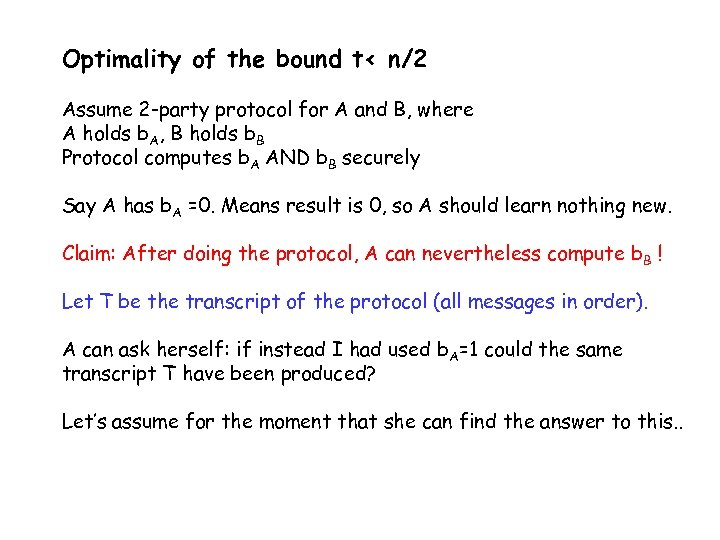Optimality of the bound t< n/2 Assume 2 -party protocol for A and B, where A holds b. A, B holds b. B Protocol computes b. A AND b. B securely Say A has b. A =0. Means result is 0, so A should learn nothing new. Claim: After doing the protocol, A can nevertheless compute b B ! Let T be the transcript of the protocol (all messages in order). A can ask herself: if instead I had used b. A=1 could the same transcript T have been produced? Let’s assume for the moment that she can find the answer to this. .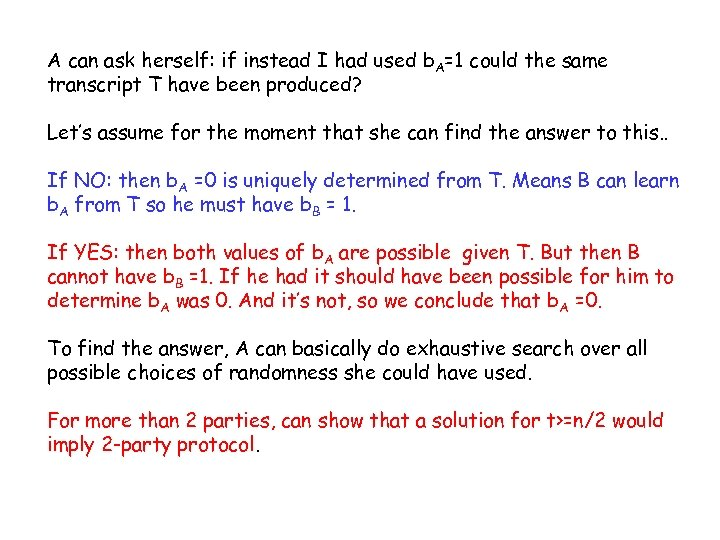A can ask herself: if instead I had used b. A=1 could the same transcript T have been produced? Let’s assume for the moment that she can find the answer to this. . If NO: then b. A =0 is uniquely determined from T. Means B can learn b. A from T so he must have b. B = 1. If YES: then both values of b. A are possible given T. But then B cannot have b. B =1. If he had it should have been possible for him to determine b. A was 0. And it’s not, so we conclude that b. A =0. To find the answer, A can basically do exhaustive search over all possible choices of randomness she could have used. For more than 2 parties, can show that a solution for t>=n/2 would imply 2 -party protocol.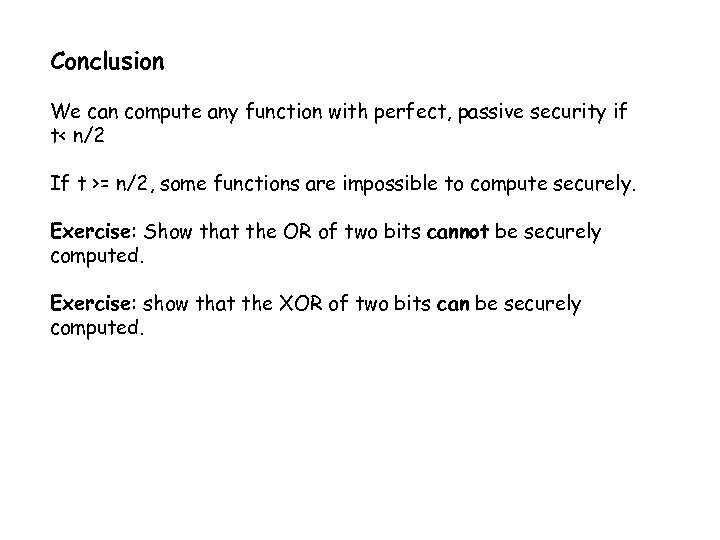Conclusion We can compute any function with perfect, passive security if t< n/2 If t >= n/2, some functions are impossible to compute securely. Exercise: Show that the OR of two bits cannot be securely computed. Exercise: show that the XOR of two bits can be securely computed.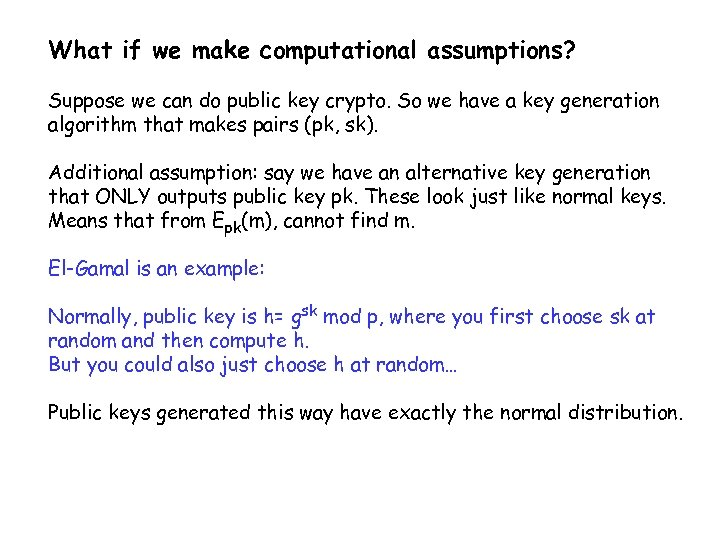What if we make computational assumptions? Suppose we can do public key crypto. So we have a key generation algorithm that makes pairs (pk, sk). Additional assumption: say we have an alternative key generation that ONLY outputs public key pk. These look just like normal keys. Means that from Epk(m), cannot find m. El-Gamal is an example: Normally, public key is h= gsk mod p, where you first choose sk at random and then compute h. But you could also just choose h at random… Public keys generated this way have exactly the normal distribution.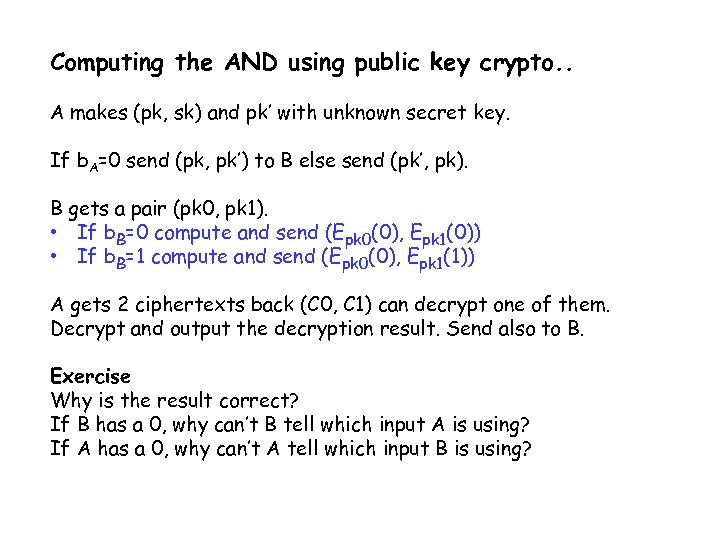Computing the AND using public key crypto. . A makes (pk, sk) and pk’ with unknown secret key. If b. A=0 send (pk, pk’) to B else send (pk’, pk). B gets a pair (pk 0, pk 1). • If b. B=0 compute and send (Epk 0(0), Epk 1(0)) • If b. B=1 compute and send (Epk 0(0), Epk 1(1)) A gets 2 ciphertexts back (C 0, C 1) can decrypt one of them. Decrypt and output the decryption result. Send also to B. Exercise Why is the result correct? If B has a 0, why can’t B tell which input A is using? If A has a 0, why can’t A tell which input B is using?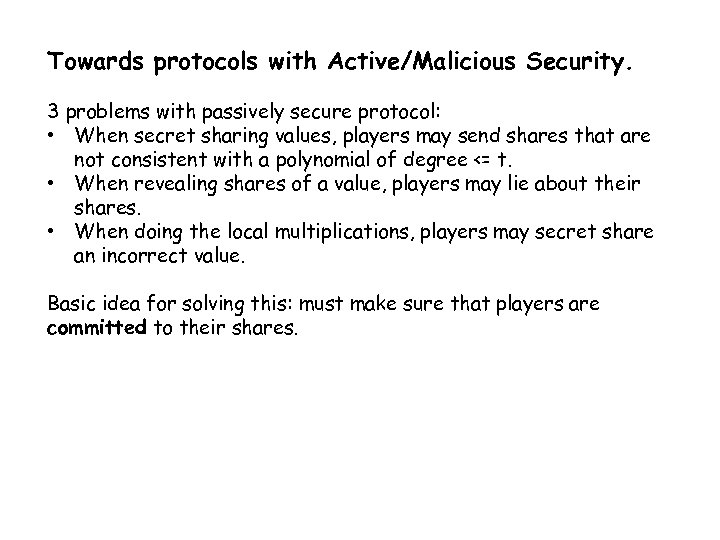Towards protocols with Active/Malicious Security. 3 problems with passively secure protocol: • When secret sharing values, players may send shares that are not consistent with a polynomial of degree <= t. • When revealing shares of a value, players may lie about their shares. • When doing the local multiplications, players may secret share an incorrect value. Basic idea for solving this: must make sure that players are committed to their shares.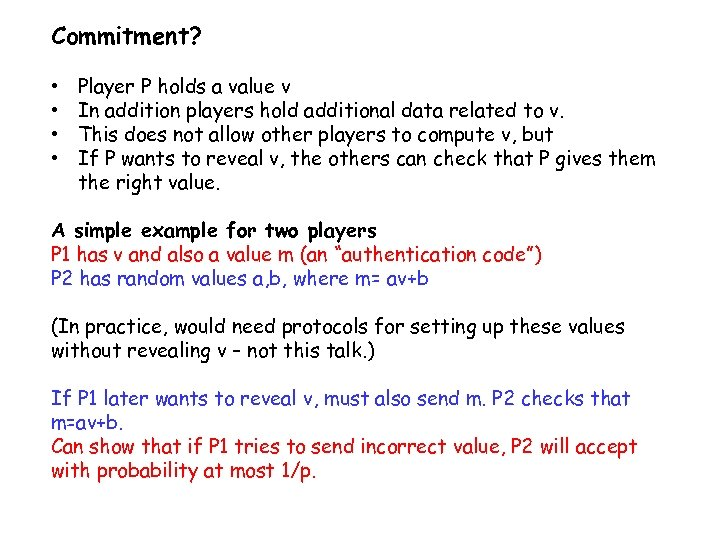Commitment? • • Player P holds a value v In addition players hold additional data related to v. This does not allow other players to compute v, but If P wants to reveal v, the others can check that P gives them the right value. A simple example for two players P 1 has v and also a value m (an “authentication code”) P 2 has random values a, b, where m= av+b (In practice, would need protocols for setting up these values without revealing v – not this talk. ) If P 1 later wants to reveal v, must also send m. P 2 checks that m=av+b. Can show that if P 1 tries to send incorrect value, P 2 will accept with probability at most 1/p.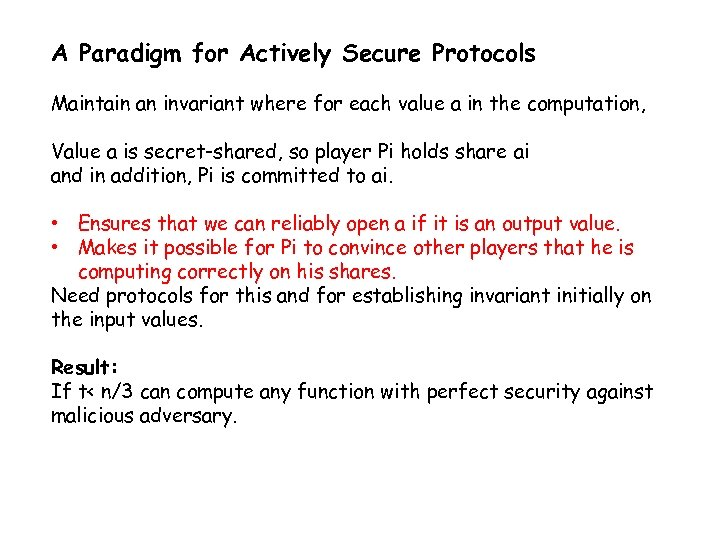A Paradigm for Actively Secure Protocols Maintain an invariant where for each value a in the computation, Value a is secret-shared, so player Pi holds share ai and in addition, Pi is committed to ai. • Ensures that we can reliably open a if it is an output value. • Makes it possible for Pi to convince other players that he is computing correctly on his shares. Need protocols for this and for establishing invariant initially on the input values. Result: If t< n/3 can compute any function with perfect security against malicious adversary.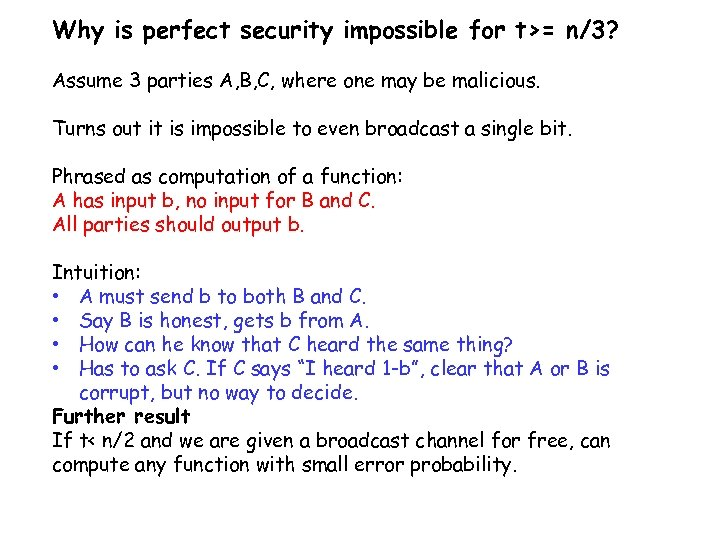Why is perfect security impossible for t>= n/3? Assume 3 parties A, B, C, where one may be malicious. Turns out it is impossible to even broadcast a single bit. Phrased as computation of a function: A has input b, no input for B and C. All parties should output b. Intuition: • A must send b to both B and C. • Say B is honest, gets b from A. • How can he know that C heard the same thing? • Has to ask C. If C says “I heard 1 -b”, clear that A or B is corrupt, but no way to decide. Further result If t< n/2 and we are given a broadcast channel for free, can compute any function with small error probability.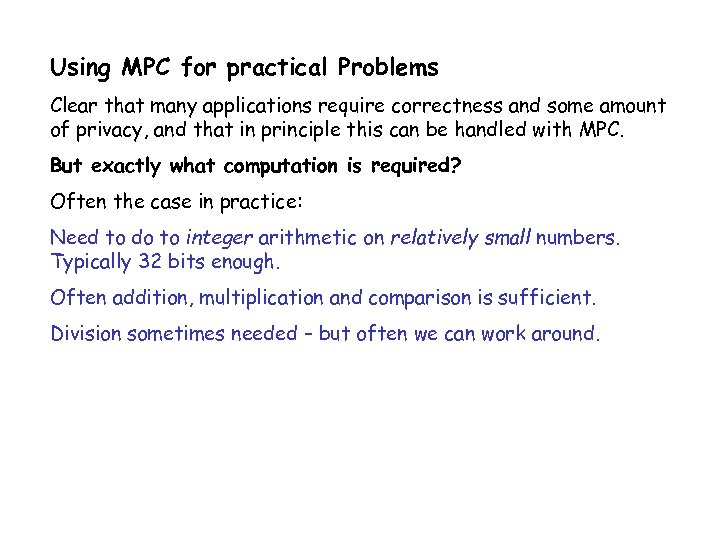Using MPC for practical Problems Clear that many applications require correctness and some amount of privacy, and that in principle this can be handled with MPC. But exactly what computation is required? Often the case in practice: Need to do to integer arithmetic on relatively small numbers. Typically 32 bits enough. Often addition, multiplication and comparison is sufficient. Division sometimes needed – but often we can work around.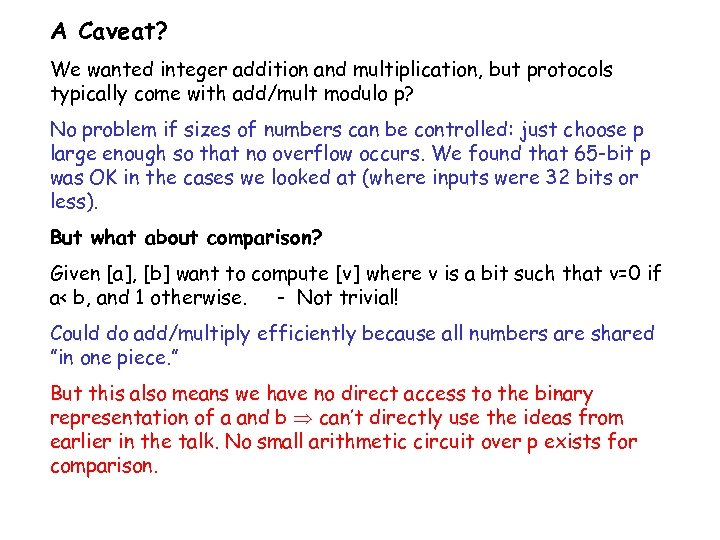A Caveat? We wanted integer addition and multiplication, but protocols typically come with add/mult modulo p? No problem if sizes of numbers can be controlled: just choose p large enough so that no overflow occurs. We found that 65 -bit p was OK in the cases we looked at (where inputs were 32 bits or less). But what about comparison? Given [a], [b] want to compute [v] where v is a bit such that v=0 if a< b, and 1 otherwise. - Not trivial! Could do add/multiply efficiently because all numbers are shared ”in one piece. ” But this also means we have no direct access to the binary representation of a and b can’t directly use the ideas from earlier in the talk. No small arithmetic circuit over p exists for comparison.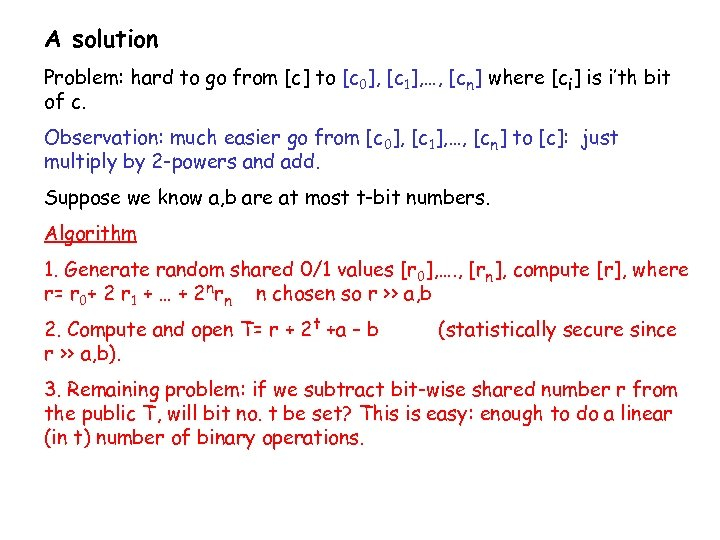A solution Problem: hard to go from [c] to [c 0], [c 1], …, [cn] where [ci] is i’th bit of c. Observation: much easier go from [c 0], [c 1], …, [cn] to [c]: just multiply by 2 -powers and add. Suppose we know a, b are at most t-bit numbers. Algorithm 1. Generate random shared 0/1 values [r 0], …. , [rn], compute [r], where r= r 0+ 2 r 1 + … + 2 nrn n chosen so r >> a, b 2. Compute and open T= r + 2 t +a – b r >> a, b). (statistically secure since 3. Remaining problem: if we subtract bit-wise shared number r from the public T, will bit no. t be set? This is easy: enough to do a linear (in t) number of binary operations.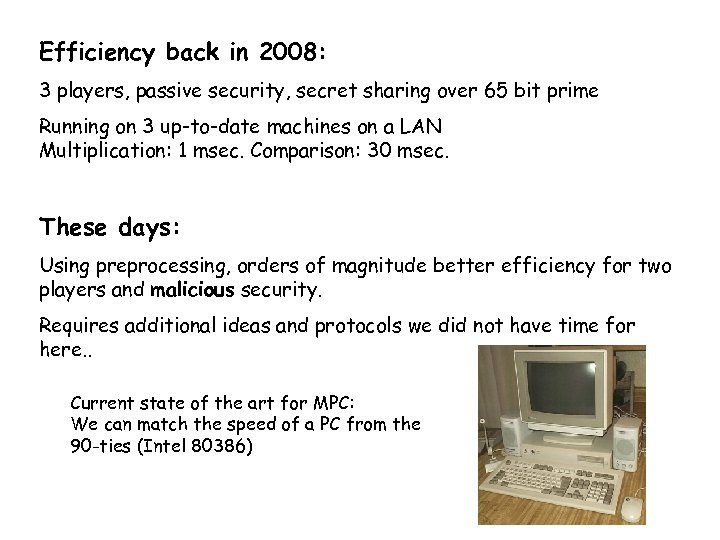Efficiency back in 2008: 3 players, passive security, secret sharing over 65 bit prime Running on 3 up-to-date machines on a LAN Multiplication: 1 msec. Comparison: 30 msec. These days: Using preprocessing, orders of magnitude better efficiency for two players and malicious security. Requires additional ideas and protocols we did not have time for here. . Current state of the art for MPC: We can match the speed of a PC from the 90 -ties (Intel 80386)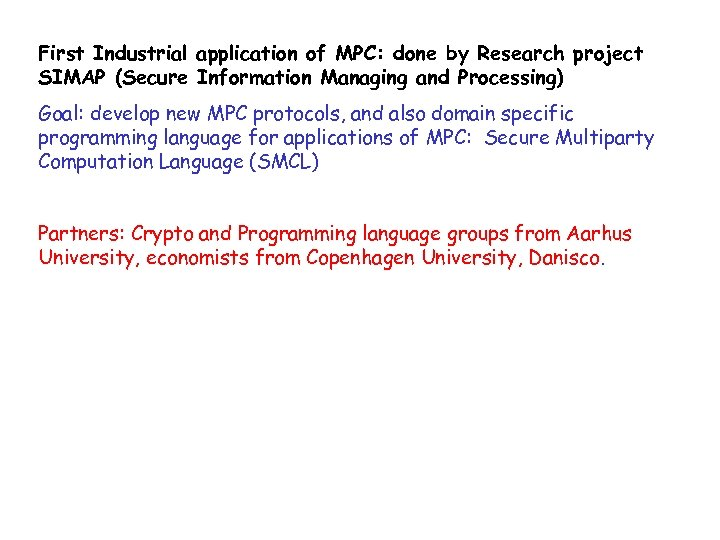First Industrial application of MPC: done by Research project SIMAP (Secure Information Managing and Processing) Goal: develop new MPC protocols, and also domain specific programming language for applications of MPC: Secure Multiparty Computation Language (SMCL) Partners: Crypto and Programming language groups from Aarhus University, economists from Copenhagen University, Danisco.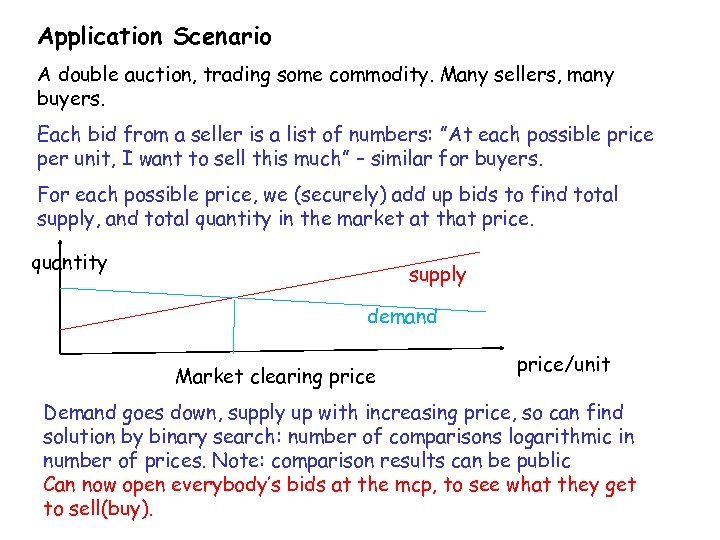Application Scenario A double auction, trading some commodity. Many sellers, many buyers. Each bid from a seller is a list of numbers: ”At each possible price per unit, I want to sell this much” – similar for buyers. For each possible price, we (securely) add up bids to find total supply, and total quantity in the market at that price. quantity supply demand Market clearing price/unit Demand goes down, supply up with increasing price, so can find solution by binary search: number of comparisons logarithmic in number of prices. Note: comparison results can be public Can now open everybody’s bids at the mcp, to see what they get to sell(buy).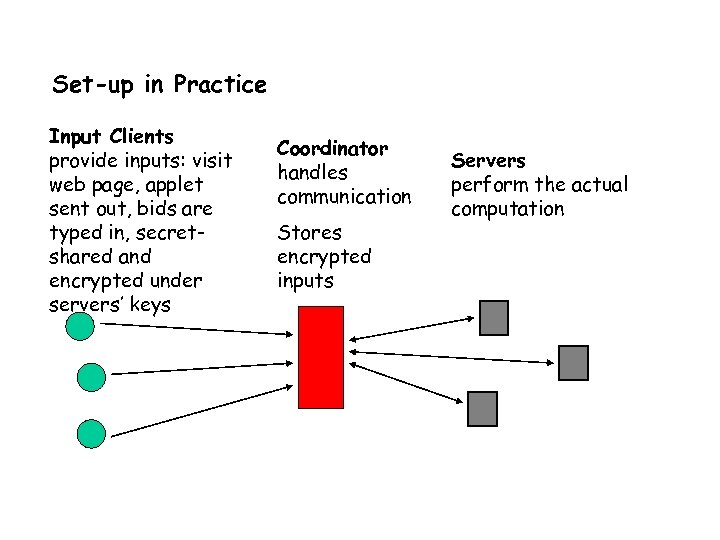Set-up in Practice Input Clients provide inputs: visit web page, applet sent out, bids are typed in, secretshared and encrypted under servers’ keys Coordinator handles communication Stores encrypted inputs Servers perform the actual computation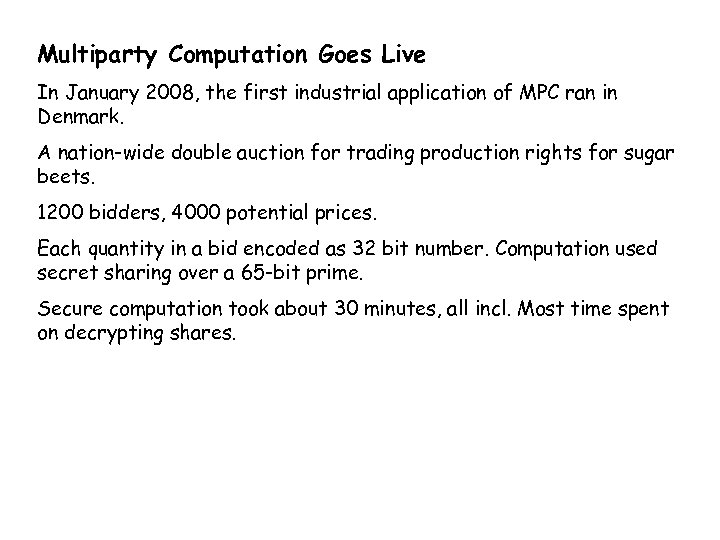Multiparty Computation Goes Live In January 2008, the first industrial application of MPC ran in Denmark. A nation-wide double auction for trading production rights for sugar beets. 1200 bidders, 4000 potential prices. Each quantity in a bid encoded as 32 bit number. Computation used secret sharing over a 65 -bit prime. Secure computation took about 30 minutes, all incl. Most time spent on decrypting shares.Why Sugar beets? Company Danisco has all sugar production in Denmark. Farmers have contracts that give them the right to deliver certain amounts of beets. Contracts can be traded between farmers, but almost no trading historically. However, EU has now reduced support for sugar beet production drastically Production can only survive if it moves to where it pays off best need for a nation wide market, natural solution is a double auction.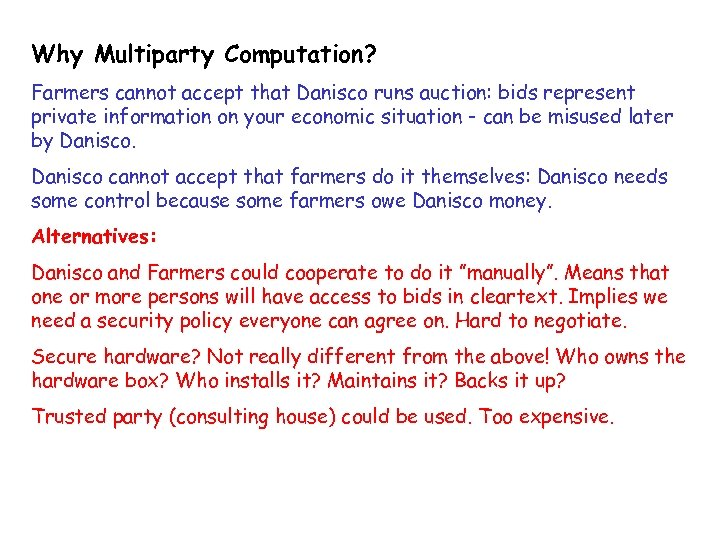Why Multiparty Computation? Farmers cannot accept that Danisco runs auction: bids represent private information on your economic situation - can be misused later by Danisco cannot accept that farmers do it themselves: Danisco needs some control because some farmers owe Danisco money. Alternatives: Danisco and Farmers could cooperate to do it ”manually”. Means that one or more persons will have access to bids in cleartext. Implies we need a security policy everyone can agree on. Hard to negotiate. Secure hardware? Not really different from the above! Who owns the hardware box? Who installs it? Maintains it? Backs it up? Trusted party (consulting house) could be used. Too expensive.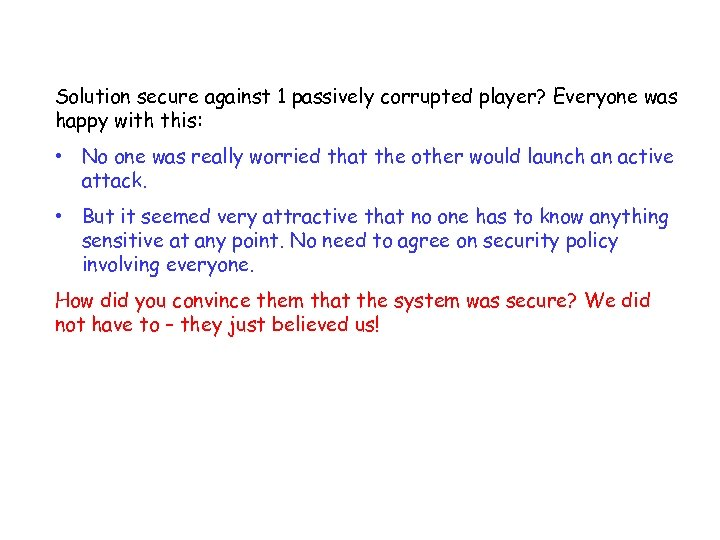Solution secure against 1 passively corrupted player? Everyone was happy with this: • No one was really worried that the other would launch an active attack. • But it seemed very attractive that no one has to know anything sensitive at any point. No need to agree on security policy involving everyone. How did you convince them that the system was secure? We did not have to – they just believed us!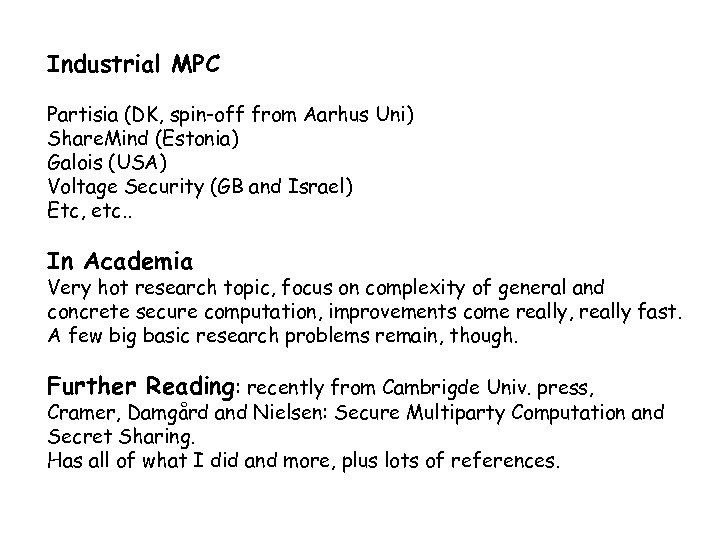Industrial MPC Partisia (DK, spin-off from Aarhus Uni) Share. Mind (Estonia) Galois (USA) Voltage Security (GB and Israel) Etc, etc. . In Academia Very hot research topic, focus on complexity of general and concrete secure computation, improvements come really, really fast. A few big basic research problems remain, though. Further Reading: recently from Cambrigde Univ. press, Cramer, Damgård and Nielsen: Secure Multiparty Computation and Secret Sharing. Has all of what I did and more, plus lots of references.